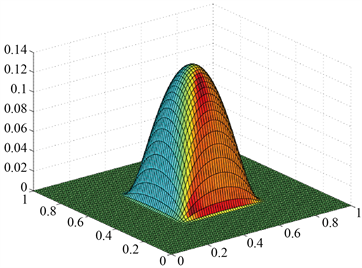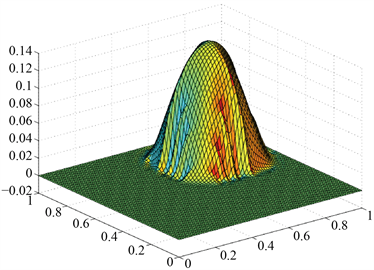﻿ 二维线性传输方程满足两个守恒律的数值格式

# 二维线性传输方程满足两个守恒律的数值格式The Numerical Scheme Satisfying Two Conservation Laws for Two Dimensional Linear Advection Equations

Abstract: In this paper, we develop an improved numerical scheme satisfying two conservation laws for two-dimensional linear advection equations, which satisfying both the numerical solution and numerical energy conservative. The numerical results show that the scheme has the super-con- vergence property away from the extreme points, and can keep the structure of the solution well.

1. 引言

2. 格式的描述

${u}_{t}+{u}_{x}+{u}_{y}=0$ (2.1)

$U{\left(u\right)}_{t}+U{\left(u\right)}_{x}+U{\left(u\right)}_{y}=0$ (2.2)

${u}_{ij}^{n}\simeq \frac{1}{{h}_{1}{h}_{2}}{\int }_{{y}_{j-\frac{1}{2}}}^{{y}_{j+\frac{1}{2}}}{\int }_{{x}_{i-\frac{1}{2}}}^{{x}_{i+\frac{1}{2}}}u\left(x,y,{t}_{n}\right)\text{d}x\text{d}y$ (2.3)

${U}_{ij}^{n}\simeq \frac{1}{{h}_{1}{h}_{2}}{\int }_{{y}_{j-\frac{1}{2}}}^{{y}_{j+\frac{1}{2}}}{\int }_{{x}_{i-\frac{1}{2}}}^{{x}_{i+\frac{1}{2}}}U\left(u\left(x,y,{t}_{n}\right)\right)\text{d}x\text{d}y$ (2.4)

$\text{A:}\left\{\begin{array}{l}{u}_{t}+{u}_{x}=0;\\ {U}_{t}+{U}_{x}=0;\\ u\left(x,y,0\right)={u}_{0}\left(x,y\right).\end{array}$ (2.5)

$\text{B:}\left\{\begin{array}{l}{u}_{t}+{u}_{y}=0;\\ {U}_{t}+{U}_{y}=0;\\ u\left(x,y,0\right)=u\left(x,y,t\right).\end{array}$ (2.6)

2.1. 守恒方程A的数值格式

$R\left(x,t;{u}_{i,j}^{n},{U}_{i,j}^{n}\right)={u}_{i,j}^{n}+{s}_{i,j}^{n}\left(x-{x}_{i}\right),\text{}x\in \left({x}_{i-\frac{1}{2}},{x}_{i+\frac{1}{2}}\right)$ (2.7)

$\frac{1}{{h}_{1}}{\int }_{{x}_{i-\frac{1}{2}}}^{{x}_{i+\frac{1}{2}}}R\left(x,t;{u}_{i,j}^{n},{U}_{i,j}^{n}\right)\text{d}x={u}_{i,j}^{n}$ (2.8)

$\frac{1}{{h}_{1}}{\int }_{{x}_{i-\frac{1}{2}}}^{{x}_{i+\frac{1}{2}}}{\left(R\left(x,t;{u}_{i,j}^{n},{U}_{i,j}^{n}\right)\right)}^{2}\text{d}x={U}_{i,j}^{n}$ (2.9)

${s}_{i,j}^{n}=\mathrm{sgn}\left({u}_{i+1,j}^{n}-{u}_{i-1,j}^{n}\right)\sqrt{\frac{12\left({U}_{i,j}^{n}-{\left({u}_{i,j}^{n}\right)}^{2}\right)}{{\left({h}_{1}\right)}^{2}}}$ (2.10)

$\left\{\begin{array}{l}{v}_{t}+{v}_{x}=0;\\ v\left(x,{y}_{j};{t}_{n}\right)=R\left(x,t;{u}_{i,j}^{n},{U}_{i,j}^{n}\right).\end{array}\text{}-\infty (2.11)

${u}_{i,j}^{A,n+1}=\frac{1}{{h}_{1}}{\int }_{{x}_{i-\frac{1}{2}}}^{{x}_{i+\frac{1}{2}}}v\left(x,{y}_{j};{t}_{n+1}\right)\text{d}x$ (2.12)

${U}_{i,j}^{A,n+1}=\frac{1}{{h}_{1}}{\int }_{{x}_{i-\frac{1}{2}}}^{{x}_{i+\frac{1}{2}}}{\left(v\left(x,{y}_{j};{t}_{n+1}\right)\right)}^{2}$ (2.13)

${u}_{i,j}^{A,n+1}={u}_{i,j}^{n}-{\lambda }_{1}\left({\stackrel{^}{f}}_{i+\frac{1}{2},j}^{n}-{\stackrel{^}{f}}_{i-\frac{1}{2},j}^{n}\right)$ (2.14)

${\stackrel{^}{f}}_{i+\frac{1}{2},j}^{n}=\frac{1}{\tau }{\int }_{{t}_{n}}^{{t}_{n+1}}v\left({x}_{i+\frac{1}{2}},{y}_{j},t\right)\text{d}t={u}_{i,j}^{n}+\frac{{s}_{i,j}^{n}}{2}\left({h}_{1}-\tau \right)$ (2.15)

${U}_{i,j}^{A,n+1}={U}_{i,j}^{n}-{\lambda }_{1}\left({\stackrel{^}{F}}_{i+\frac{1}{2},j}^{n}-{\stackrel{^}{F}}_{i-\frac{1}{2},j}^{n}\right)$ (2.16)

$\begin{array}{c}{\stackrel{^}{F}}_{i+\frac{1}{2},j}^{n}=\frac{1}{\tau }{\int }_{{t}_{n}}^{{t}_{n+1}}{\left(v\left({x}_{i+\frac{1}{2}},{y}_{j},t\right)\right)}^{2}\text{d}t\\ ={\left({u}_{i,j}^{n}\right)}^{2}+{u}_{i,j}^{n}{s}_{i,j}^{n}\left({h}_{1}-\tau \right)+{\left({s}_{i,j}^{n}\right)}^{2}{\left({h}_{1}\right)}^{2}\frac{4{\lambda }_{1}{}^{2}-6{\lambda }_{1}+3}{12}\end{array}$ (2.17)

2.2. 守恒方程B的数值格式

$R\left(y;{u}_{i,j}^{A,n\text{+}1},{U}_{i,j}^{A,n\text{+}1}\right)={u}_{i,j}^{A,n\text{+}1}+{\sigma }_{i,j}^{n\text{+}1}\left(y-{y}_{j}\right),\text{}y\in \left({y}_{j-\frac{1}{2}},{y}_{j+\frac{1}{2}}\right)$ (2.18)

$\left\{\begin{array}{l}\frac{1}{{h}_{2}}{\int }_{{y}_{j-\frac{1}{2}}}^{{y}_{j+\frac{1}{2}}}R\left(y;{u}_{i,j}^{A,n\text{+}1},{U}_{i,j}^{A,n\text{+}1}\right)\text{d}y={u}_{i,j}^{A,n\text{+}1},\\ \frac{1}{{h}_{2}}{\int }_{{y}_{j-\frac{1}{2}}}^{{y}_{j+\frac{1}{2}}}{\left(R\left(y;{u}_{i,j}^{A,n\text{+}1},{U}_{i,j}^{A,n\text{+}1}\right)\right)}^{2}\text{d}y={U}_{i,j}^{A,n\text{+}1},\end{array}$ (2.19)

${\sigma }_{i,j}^{n\text{+}1}=\mathrm{sgn}\left({u}_{i,j+1}^{A,n\text{+}1}-{u}_{i,j-1}^{A,n\text{+}1}\right)\sqrt{\frac{12\left({U}_{i,j}^{A,n\text{+}1}-{\left({u}_{i,j}^{A,n\text{+}1}\right)}^{2}\right)}{{\left({h}_{2}\right)}^{2}}}$ (2.20)

$\left\{\begin{array}{l}{v}_{t}+{v}_{y}=0;\\ v\left({x}_{i},y;{t}_{n}\right)=R\left(y,t;{u}_{i,j}^{A,n\text{+}1},{U}_{i,j}^{A,n\text{+}1}\right).\end{array}\text{}-\infty (2.21)

$\left\{\begin{array}{l}{u}_{i,j}^{n+1}={u}_{i,j}^{A,n+1}-{\lambda }_{2}\left({\stackrel{^}{g}}_{i,j+\frac{1}{2}}^{n}-{\stackrel{^}{g}}_{i,j-\frac{1}{2}}^{n}\right),\\ {U}_{i,j}^{n+1}={U}_{i,j}^{A,n+1}-{\lambda }_{2}\left({\stackrel{^}{G}}_{i,j+\frac{1}{2}}^{n}-{\stackrel{^}{G}}_{i,j-\frac{1}{2}}^{n}\right),\end{array}$ (2.22)

${\stackrel{^}{g}}_{i,j+\frac{1}{2}}^{n\text{+}1}=\frac{1}{\tau }{\int }_{{t}_{n}}^{{t}_{n+1}}v\left({x}_{i},{y}_{j+\frac{1}{2}},t\right)\text{d}t={u}_{i,j}^{A,n\text{+}1}+\frac{{\sigma }_{i,j}^{n\text{+}1}}{2}\left({h}_{2}-\tau \right),$ (2.23)

$\begin{array}{c}{\stackrel{^}{G}}_{i,j+\frac{1}{2}}^{n\text{+}1}=\frac{1}{\tau }{\int }_{{t}_{n}}^{{t}_{n+1}}{\left(v\left({x}_{i},{y}_{j+\frac{1}{2}},t\right)\right)}^{2}\text{d}t\\ ={\left({u}_{i,j}^{A,n\text{+}1}\right)}^{2}+{u}_{i,j}^{A,n\text{+}1}{\sigma }_{i,j}^{n\text{+}1}\left({h}_{2}-\tau \right)+{\left({\sigma }_{i,j}^{n\text{+}1}\right)}^{2}{\left({h}_{2}\right)}^{2}\frac{4{\lambda }_{2}{}^{2}-6{\lambda }_{2}+3}{12}\end{array}$ (2.24)

${u}_{i,j}^{n+1}={u}_{i,j}^{n}-{\lambda }_{1}\left({\stackrel{^}{f}}_{i+\frac{1}{2},j}^{n}-{\stackrel{^}{f}}_{i-\frac{1}{2},j}^{n}\right)-{\lambda }_{2}\left({\stackrel{^}{g}}_{i,j+\frac{1}{2}}^{n}-{\stackrel{^}{g}}_{i,j-\frac{1}{2}}^{n}\right),$ (2.25)

${U}_{i,j}^{n+1}={U}_{i,j}^{n}-{\lambda }_{1}\left({\stackrel{^}{F}}_{i+\frac{1}{2},j}^{n}-{\stackrel{^}{F}}_{i-\frac{1}{2},j}^{n}\right)-{\lambda }_{2}\left({\stackrel{^}{G}}_{i,j+\frac{1}{2}}^{n}-{\stackrel{^}{G}}_{i,j-\frac{1}{2}}^{n}\right).$ (2.26)

3. 格式的可行性

${U}_{i,j}^{0}\ge {\left({u}_{i,j}^{0}\right)}^{2}$ (3.1)

$\left\{\begin{array}{l}{u}_{i,j}^{A,n+1}=\frac{1}{{h}_{1}}{\int }_{{x}_{i-\frac{1}{2}}}^{{x}_{i+\frac{1}{2}}}v\left(x,{y}_{j};{t}_{n+1}\right)\text{d}x,\\ {U}_{i,j}^{A,n+1}=\frac{1}{{h}_{1}}{\int }_{{x}_{i-\frac{1}{2}}}^{{x}_{i+\frac{1}{2}}}{\left(v\left(x,{y}_{j};{t}_{n+1}\right)\right)}^{2}\text{d}x,\end{array}$ (3.2)

$\frac{1}{{h}_{1}}{\int }_{{x}_{i-\frac{1}{2}}}^{{x}_{i+\frac{1}{2}}}U\left(v\left(x,{y}_{j},{t}_{n+1}\right)\right)\text{d}x\ge U\left(\frac{1}{{h}_{1}}{\int }_{{x}_{i-\frac{1}{2}}}^{{x}_{i+\frac{1}{2}}}v\left(x,{y}_{j},{t}_{n+1}\right)\right)\text{d}x,$ (3.3)

${U}_{i,j}^{A,n+1}\ge {\left({u}_{i,j}^{A,n+1}\right)}^{2}$ (3.4)

${U}_{i,j}^{n+1}\ge {\left({u}_{i,j}^{n+1}\right)}^{2}$ (3.5)

4. 数值算例

$u\left(x,y,0\right)=\mathrm{sin}\left(2\pi \left(x+y\right)\right),\text{}0\le x\le 1$ (4.1)Table 1. The error and convergence order of interval [0, 1] ×[0, 1]Table 2. The error and convergence order of interval Ω

$\left\{\begin{array}{l}u\left(x,y,0\right)=\mathrm{exp}\left(-\frac{1}{1-16{\left(x-\frac{1}{2}\right)}^{2}}\right)×\mathrm{exp}\left(-\frac{1}{1-16{\left(y-\frac{1}{2}\right)}^{2}}\right)\\ \left(x,y\right)\in \left(\frac{1}{4},\frac{3}{4}\right)×\left(\frac{1}{4},\frac{3}{4}\right)\\ \\ u\left(x,y,0\right)=0,\text{}\left(x,y\right)\in \left(\frac{1}{4},\frac{3}{4}\right)×\left(\frac{1}{4},\frac{3}{4}\right)\end{array}$ (4.2)

5. 结论(a) t = 0时刻的解(b) t = 0.2时刻的数值解

Figure 1. Results of example 4.2 at different time

 Cui, Y. and Mao, D. (2012) Error Self-Canceling of a Difference Scheme Maintaining Two Conservation Laws for Linear Advection Equation. Mathematics of Computation, 81, 715-741.
https://doi.org/10.1090/S0025-5718-2011-02523-8

 Cui, Y. and Mao, D. (2007) Numerical Method Satisfying the First Two Conservation Laws for the Korteweg-De Vries Equation. Journal of Computational Physics, 227, 376-399.
https://doi.org/10.1016/j.jcp.2007.07.031

 崔艳芬. 线性传输方程和KdV方程的满足两个守恒律的差分格式[D]: [博士学位论文]. 上海: 上海大学, 2008.

 李红霞. 一维守恒型方程(组)的熵耗散格式[D]: [博士学位论文]. 上海: 上海大学, 2005.

 王志刚. 线性传输方程满足多个守恒律的差分格式[D]: [硕士学位论文]. 上海: 上海大学, 2005.

 王志刚, 茅德康. 线性传输方程满足三个守恒律的差分格式[J]. 上海大学学报(自然科学版), 2006(6): 588-592.

 Le Veque, R.J. (2002) Finite Volume Methods for Hyperbolic Problems. Syndicate of the University of Cambridge Press, Cambridge.
https://doi.org/10.1017/CBO9780511791253

 李立康, 於崇华, 朱政华. 微分方程数值解法[M]. 上海: 复旦大学出版社, 1999.

Top